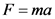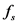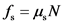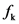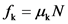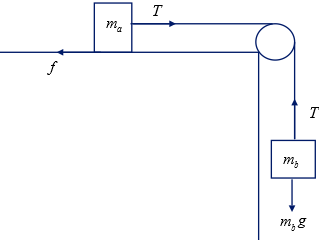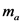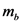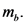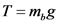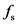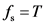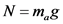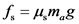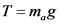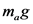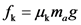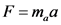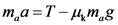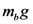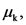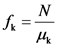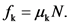# What minimum value of ma will keep the system from starting to move?

The coefficient of static
friction between mass ma and the table is 0.40, whereas the
coefficient of kinectic friciton is .30.
A) what minimum value of ma will keep the system from starting to
move?
B) what value(s) of ma will keep the system moving at a constand
speed?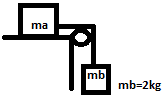General guidance

Concepts and reason
The concepts required to solve the given problem are Newton’s second law of motion, static frictional force and kinetic frictional force.
Initially, draw a free body diagram for each mass by using given data. Then, determine the mass of the mass placed on the table by knowing tension in the rope. Finally, determine the mass of the mass placed on the table when it is moving with constant speed by using Newton’s second law of motion and by obtaining expression for net force on the mass on the table.

Fundamentals

The net force F on object is equal to product of mass m of the object and acceleration a of the object.

The acceleration of an object is equal to zero when the object moving with a constant speed.
The static frictional force is the minimum force required to move an object. The expression for static frictional force is given as follows:

Here, N is the normal force and is the coefficient of static friction.
The kinetic frictional force on an object is given by following expression.

Here, is the coefficient of kinetic friction.

Step-by-step

Step 1 of 2

(A)
Draw the following free body diagram for each mass as follows:

Here, is the mass of the block on table, is the mass of the block hanged, T in the tension in the string, g is the acceleration due to gravity and f is the frictional force.
From the figure, the tension T in the string is equal to weight of block

The static frictional force is equal to tension T in the rope.

The static frictional force on the block on the table is given by following expression.

The normal force on the mass is equal to weight of the mass

Substitute in the above equation to solve for static frictional force.

Substitute and in the above equation to solve for

Substitute 2 kg for and 0.40 for in the above equation.

Part A
The minimum value for mass is 5 kg.

The minimum force required to move the block of mass is equal to static frictional force on the block that is equal to tension in the rope. Thus, the frictional force on the block is equal to tension in the rope.

Do not take the expression for tension in the rope as because the correct expression is .

Determine the mass of the block on the table to move the system with constant speed by using Newton’s second law of motion and by obtaining net force on the block on the table.

Step 2 of 2

(B)
From the free body diagram, the net force on the block on the table is given by following expression.

Here, is the kinetic frictional force.
The kinetic frictional force on the block is given by following expression.

Substitute for N in the above equation.

According to Newton’s second law of motion, the net force on the block is given by following expression.

Substitute and in the above equation .

Substitute 0 for a, and for T in the above equation to solve for mass of block on table.

Substitute 0.30 for and 2 kg for in the above equation.

Part B
The minimum value for mass is 6.67 kg.

Since the block is moving with constant speed, the acceleration of the block must be equal to zero.

Do not take the expression for kinetic friction as because the correct expression is

Part A
The minimum value for mass is 5 kg.

Part B
The minimum value for mass is 6.67 kg.

Part A
The minimum value for mass is 5 kg.

Part B
The minimum value for mass is 6.67 kg.

F=ma
f = .N
fa=4N
144
T=m,g
fs=1
f = .N
N=m,8
N=m,8
f = .N
fs = umg
fs = umg
T=m,g
fs=1
um.g=mg
2 kg m = 0.4 = 5 kg
T=m,g
T=m,g
F=T-
fa=4N
f = 4mg
F=ma
F=ma
f = 4mg
F=T-
ma=T-4mg
m.(0=m,g-4mg
m. – 2kg ma 0.3 = 6.67 kg
fx = 44N.
We were unable to transcribe this image